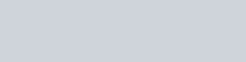# kVA to VA – Calculator, How to convert in one step, How many 1 kVA to VA

To convert from kVA to VA you simply multiply the kVA x 1000 or use the quick calculator in this article.

Here you will also find the conversion formula , with some descriptive examples , the main equivalences in a quick table and finally the steps to perform the calculation .

Mas información:

## Formula:## How to convert from kVA to VA in 1 only step.

### Step 1:

Multiply kVA by 1000. Example, if you have a microwave that consumes 1.2kVA, you must multiply 1.2kVA per 1000, the result will be: 1200VA.

## Definition VA and S (kVA):

VA: A Volt-ampere, commonly referred to as a VA, is commonly used as a unit of power in obtaining the electrical capacity of circuit breakers, uninterrupted power supplies and wirings.

VA is larger than Watts because loads are inductive such as motors, discharge lighting, reactors and more current is required to keep the magnetic field energized than is -turned into heat (Watts).

Inductive devices or loads such,. as tansformers and motors having power factor less than 1.0 are generally rated in VA.

kVA=It is the same power previously named (VA) only that is a thousand times greater.